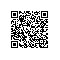# 算法学习之路|数位dp简要分析

1.由于千位是2，首先求出[0,2000)中满足条件的个数，因为此时个十百位可以任意取值，不受上界的限制。

2.之后由于千位已经到达最高，要改为考虑百位，此时百位受到上界的限制，所以之后需要求出[2000,2300)中马满足条件的个数，此时十位和个位不受限制。

3.当百位是3时，十位又受到限制…以此类推。


#include<stdio.h>
#include<algorithm>
#include<iostream>
#define n 20
using namespace std;
typedef long long ll;
ll dp[n][state];//state作为状态，不同的题目可能有不同的状态个数，有时甚至需要改变dp的维数，第一维代表数的长度
ll dd[n];//将数的每一位存储到数组
ll dfs(int pos,int state,bool lead,bool limit)
/*pos表示当前的数位，此处pos=0表示个位，pos=1表示十位，以此类推
state表示状态参数，也可以有多个状态参数
limit表示当前pos位是否受限）*/
{
if(pos==-1)//表示已经搜索结束
return 1;//返回并不确定，结合实际情况改变
return dp[pos][state];
int now=limit?dd[pos]:9;//now用以确定搜索终点，不受限就是9，受限就是当前pos位的数值
ll ans=0;
for(int i=0;i<=now;i++)//注意边界
{
}
dp[pos][state]=ans;
return ans;
}


## hdu2089

ac代码：

#include<stdio.h>
#include<iostream>
#include<algorithm>
#include<string.h>
using namespace std;
typedef long long ll;
ll dp;
ll dd;
ll dfs(int pos,int pre,bool limit)//这里前导零不影响结果，不需要lead   pre表示pos位前一位的数字
{
if(pos==-1)
return 1;//进行到这里说明之前位已经全部通过，返回1
if(!limit&&dp[pos][pre]!=-1)
return dp[pos][pre];
int now=limit?dd[pos]:9;
ll ans=0;
for(int i=0;i<=now;i++)
{
if(i==4||i==2&&pre==6)continue;//是不吉利的数就跳过
ans+=dfs(pos-1,i,limit&&(i==dd[pos]));
}
if(!limit)
dp[pos][pre]=ans;
return ans;
}
ll build(int n)//计算从1-n的个数
{
int k=0;
while(n)
{
dd[k++]=n%10;
n/=10;
}
return dfs(k-1,0,true);
}
int main()
{
memset(dp,-1,sizeof(dp));//初始化
int a,b;
while(scanf("%d%d",&a,&b),a+b)
{
printf("%ld\n",build(b)-build(a-1));
}
return 0;
}使用钉钉扫一扫加入圈子
+ 订阅